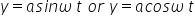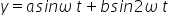# Harmonic and Non-harmonic Oscillation

Harmonic oscillation is the repetitive movement of an object or system about a fixed point or equilibrium position in response to a restoring force. It is a type of periodic motion that can be modeled mathematically as a sinusoidal function. Examples of harmonic oscillation include a pendulum swinging back and forth, a mass on a spring bouncing up and down, and the oscillation of an electric circuit.

Harmonic oscillation is that oscillation which can be expressed in terms of single harmonic function (i.e. sine or cosine function). Example :Non-harmonic oscillation refers to any type of oscillation that does not follow a simple harmonic motion (SHM) pattern. In SHM, the restoring force is proportional to the displacement from the equilibrium position, and the motion is sinusoidal. In contrast, non-harmonic oscillations can have more complex patterns of motion and may be caused by forces that are not proportional to the displacement. Examples of non-harmonic oscillations include chaotic systems, damped oscillations, and forced oscillations.

Non-harmonic oscillation is that oscillation which can not be expressed in terms of single harmonic function. It is a combination of two or more

than two harmonic oscillations. Example :Related Keywords